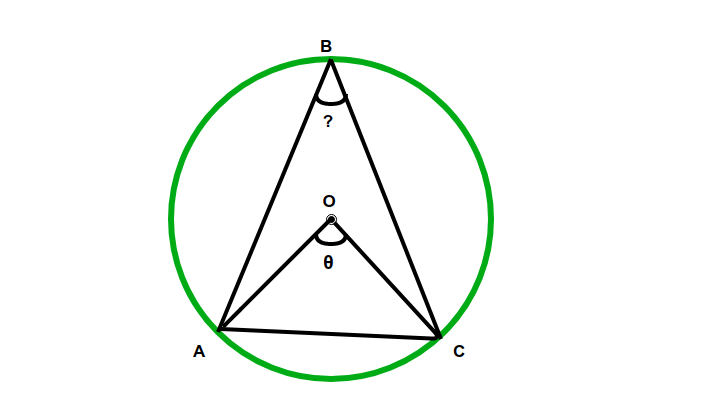Open in App
Not now

# Program to calculate angle on circumference subtended by the chord when the central angle subtended by the chord is given

• Difficulty Level : Hard
• Last Updated : 12 Oct, 2022

Given a circle having a chord and an angle subtended by chord on center of the circle. The task here is to find the measure of the angle subtended by given chord on the circumference.Examples:

Input:= 90Output: ABC = 45.00 degreesInput:= 65Output: ABC = 32.50 degrees

Approach:

• Let AC be an chord of a circle with centre O, and let C be any point on the circumference anywhere.
• Let, angle AOC(on center) is the given.
• So angle should be on the circumference,
angle ABC = angle AOC/2

An angle at the circumference of a circle is the half angle at the center subtended by the same chord.

Below is the implementation of the above approach:

## C++

 // C++ Program to calculate angle// on the circumference subtended// by the chord when the central angle// subtended by the chord is given#include using namespace std; float angleOncirCumference(float z){    return (z / 2);} // Driver codeint main(){     // Angle on center    float angle = 65;     float z = angleOncirCumference(angle);     cout << "The angle is " << (z) << " degrees";     return 0;} // This code is contributed by jit_t

## Java

 // Java Program to calculate angle on the circumference// subtended by the chord when the central angle// subtended by the chord is given class GFG {     static float angleOncirCumference(float z)    {        return (z / 2);    }     // Driver code    public static void main(String[] args)    {         // Angle on center        float angle = 65;         float z = angleOncirCumference(angle);         System.out.println("The angle is "                           + z + " degrees");    }}

## Python3

 # Python3 Program to calculate angle# on the circumference subtended# by the chord when the central angle# subtended by the chord is givendef angleOncirCumference(z):     return (z / 2); # Driver code # Angle on centerangle = 65; z = angleOncirCumference(angle); print("The angle is", (z), "degrees"); # This code is contributed by Rajput-Ji

## C#

 // C# Program to calculate angle on the circumference// subtended by the chord when the central angle// subtended by the chord is givenusing System;     public class GFG{     static float angleOncirCumference(float z)    {        return (z / 2);    }     // Driver code    public static void Main(String[] args)    {         // Angle on center        float angle = 65;         float z = angleOncirCumference(angle);         Console.WriteLine("The angle is "                        + z + " degrees");    }} // This code is contributed by Rajput-Ji

## Javascript

 

Output:

The angle is 32.5 degrees

Time Complexity: O(1)

Auxiliary Space: O(1)

My Personal Notes arrow_drop_up